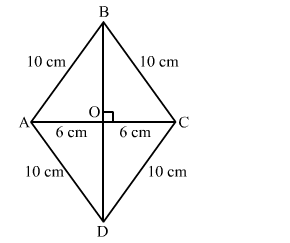# In a rhombus of side 10 cm, one of the diagonals is 12 cm long. The length of the second diagonal isQuestion:

In a rhombus of side 10 cm, one of the diagonals is 12 cm long. The length of the second diagonal is
(a) 20 cm
(b) 18 cm
(c) 16 cm
(d) 22 cm

Solution:

(c) 16 cmLet ABCD be the rhombus with diagonals AC and BD intersecting each other at O.
Also, diagonals of a rhombus bisect each other at right angles.
If AC =12 cm, AO = 6 cm
Applying Pythagoras theorem in right-angled triangle AOB. we get:

$A B^{2}=A O^{2}+B O^{2}$

$\Rightarrow B O^{2}=A B^{2}-A O^{2}$

$\Rightarrow B O^{2}=10^{2}-6^{2}=100-36=64$

$\Rightarrow B O=\sqrt{64}=8$

$\Rightarrow B D=2 \times B O=2 \times 8=16 \mathrm{~cm}$

Hence, the length of the second diagonal BD is 16 cm.